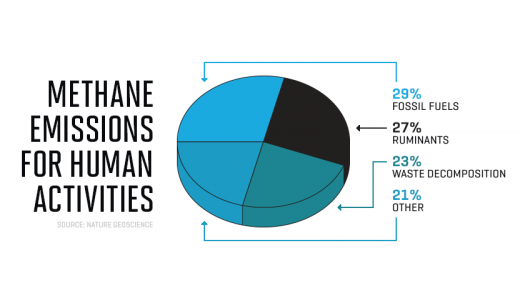# What Do You Think You Know About Methane

10 Questions | Total Attempts: 115SettingsMethane is a chemical compound that has the chemical formula CH4. The compound is a group-14 hybrid and it is also the most basic alkane known. On that note, how about some questions?

• 1.
Natural methane is found both below ground and under the?
• A.

Sea floor

• B.

Three

• C.

Earth

• D.

Atmosphere

• 2.
When it reaches the surface and the atmosphere, it is known as?
• A.

Atmosphere

• B.

Earth

• C.

Hydrogen

• D.

Atmospheric methane

• 3.
Methane is used as a fuel for ovens, homes, water heaters and?
• A.

Kilns

• B.

Automobiles

• C.

Turbines

• D.

All of the above

• 4.
Methane was first scientifically identified by?
• A.

Italian

• B.

Switzerland

• C.

Franklin

• D.

A and B only

• 5.
Methane is a tetrahedral molecule with four equivalent?
• A.

C-H bonds

• B.

Alkaline

• C.

PH

• D.

None of the above

• 6.
At room temperature and standard pressure, methane is a?
• A.

Colorless

• B.

Odorless gas

• C.

Alkaline earth metal

• D.

A and B only

• 7.
Solid state exists in several modifications, presently, the number of known solid methane is?
• A.

5

• B.

6

• C.

8

• D.

9

• 8.
The primary chemical reactions of methane are?
• A.

Combustion

• B.

Steam reforming

• C.

Halogenation

• D.

All of the above

• 9.
In general, methane reactions are difficult to?
• A.

Control

• B.

Contain

• C.

Tame

• D.

All of the above

• 10.
The species M* signifies an energetic third body, from which energy is transferred during a?
• A.

Molecular collision

• B.

Chemical reaction

• C.

Hydrocarbon

• D.

None of the above

Related TopicsBack to top